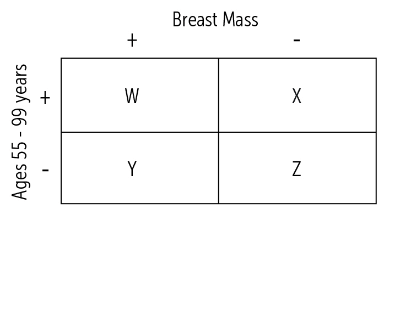USMLE-Rx Step 1 Practice Q's

# USMLE-Rx Step 1 Qmax Challenge #1557

Check out today’s Step 1 Qmax Question Challenge.

Know the answer? Post it below! Don’t forget to check back for an update with the correct answer and explanation (we’ll post it in the comments section below).A 50-year-old woman presents for a routine physical examination. She has recently retired from her position as a statistics professor. During her visit she complains about a breast lump near her left areola. A breast examination is concerning for a smooth mass near the patient described location. The physician performs a cross sectional study looking at all women aged 50-99 years in his town and determines the prevalence of all breast masses of this age group.

Using the letters in the figure, which of the following equations would help the physician accurately describe the prevalence of breast masses to this statistician?

A. (W + X) / (W + X + Y + Z)
B. (W + Y)/(W + X + Y + Z)
C. W/(W + X + Y + Z)
D. W/(X + Y + Z)
E. W/(X + Z)

———————–

Want to know the ‘bottom line?’ Purchase a USMLE-Rx Subscription and get many more features, more questions, and passages from First Aid, including images, references, and other facts relevant to this question.

This practice question is an actual question from the USMLE-Rx Step 1 Qmax test bank. For more USMLE Step 1 prep, subscribe to our First Aid Step 1 Flash Facts and First Aid Step 1 Express Videos video series. Score the best deal on all three products as a bundle with USMLE-Rx 360 Step 1.

### 4 replies »

1.Dalianne says:

B?
Prevalece = (# of patients with disease in the population) / (total population).

2.Shree says:

B

3.DR PATEL says:

C

4.firstaidrjs says:

The correct answer is B. The prevalence is the number of individuals with a disease in a given population at risk for that particular disease at a given time. Prevalence is estimated by test results but is not a measure of a test’s validity. In the chart, the prevalence can also be determined by calculating the number of true-positive plus false-negative results divided by the total number of individuals in the selected population: (TP + FN)/(TP + FP + FN + TN).

A is not correct. (TP + FP)/(TP + FP + FN + TN): this term represents the incidence of positive test results.

C is not correct. TP/(TP + FP + FN + TN): this represents true-positive results divided by the total number of patients. This would be the percentage of true-positive results of all tested, but it is not used very often.

D is not correct. TP/(FP + FN + TN): this represents true-positive results divided by the total number of patients tested less those with true-positive results, and would not be a meaningful calculation.

E is not correct. TP/(FP + TN): this represents the number of true-positive results over the total number of patients without disease. This would not be a meaningful calculation.